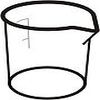#### You may also like### Brimful

Can you find the volumes of the mathematical vessels?

# Brimful 2

## Part 1:

The volume generated by rotation about the $y$-axis can be found by the method of shells or the method of discs.

The method of shells is based upon filling the solid of revolution with an infinite number of thin cylindrical shells. The volume of each shell for the revolution about the $y$-axis of the curve $y = f(x)$, is equal to its circumference $2 \pi x$ multiplied by a thickness $\mathrm{d}x$ multiplied by its height $f(x)$. By allowing the thickness of each shell to approach 0 ($\mathrm{d}x \to 0$) and summing all shells we obtain the definite integral:
$$\textrm{[Volume]} = \int 2 \pi \ x \ f(x) \ \mathrm{d}x$$

Here we shall use the conventional method of discs; we split the solid up into a series of thins slices and by rotating a slice about the y axis we will generate a cylinder of volume $\pi f(y)^2\ \mathrm{d}y$. Letting $\mathrm{d}y \to 0$ and summing all discs we obtain the definite integral:
$$\textrm{[Volume]} = \pi \int f(y)^2 \ \mathrm{d}y$$

### Curve 1:

$y = \frac{-1}{x}$

$f(y)^2 = y^{-2}$

Limits of integration are $y=-\infty \to y=-1$

$\displaystyle \textrm{[Volume]} = \pi \int_{- \infty}^{-1} y^{-2} \ \mathrm{d}y = - \pi$

We can therefore fill this vessel.

### Curve 2:

$y = \frac{-1}{x^2}$

$f(y)^2 = \frac{-1}{y}$

Limits of integration are $y=-\infty \to y=-1$

$\displaystyle \textrm{[Volume]} = \pi \int_{- \infty}^{-1} \frac{-1}{y} \ \mathrm{d}y = \infty$

We will therefore never be able to fill this vessel.

### Curve 3:

$y = \frac{1}{x^{1.5}}$

$f(y)^2 = y^{\frac{-4}{3}}$

Limits of integration are $y=-\infty \to y= -1$

$\displaystyle \textrm{[Volume]} = \pi \int_{- \infty} ^{-1} y^{\frac{-4}{3}} \ \mathrm{d}y = 3\pi$

We can therefore fill this vessel.

### Curve 4:

$y = \ln(x)$

$f(y)^2 = e^{2y}$

Limits of integration are $y=-\infty \to y=-1$

$\displaystyle \textrm{[Volume]} = \pi \int_{- \infty}^{-1} e^{2y} \ \mathrm{d}y = \pi \frac{e^{-2}}{2}$

We can therefore fill this vessel

## Extension 1:

### Curve 1: $y = \frac{-1}{x}$

At $x = 1$, $y = -1$
At $x = 10^{-4}$, $y = -10^4$

$\displaystyle \textrm{[Volume]}= \pi \int_{-10^4}^{-1}y^{-2} \ \mathrm{d}y$

$\textrm{[Volume of flask]} = 3.14 \mathrm{\ cm^3}$

### Curve 2: $y = \frac{-1}{x^2}$

At $x = 1$, $y = -1$
At $x = 10^{-4}$, $y = -10^8$

$\displaystyle \textrm{[Volume]} = \pi \int_{-10^8}^{-1} -y^{-1} \ \mathrm{d}y$

$\textrm{[Volume of flask]} = 57.9 \mathrm{\ cm^3}$

### Curve 3: $y = \frac{1}{x^{1.5}}$

At $x = 1$, $y = -1$
At $x = 10^{-4}$, $y = 10^6$

$\displaystyle \textrm{[Volume]} = \pi \int_{-1}^{10^6} y^{\frac{-4}{3}} \ \mathrm{d}y$

$\textrm{[Volume of flask]} = 9.33 \mathrm{\ cm^3}$

### Curve 4: $y = \ln(x)$

At $x = 1$, $y = 0$
At x = $10^{-4}$, y =$\ln(10^{-4})$

$\displaystyle \textrm{[Volume]} = \pi \int_{ln(10^{-4})}^{0}e^{2y} \ \mathrm{d}y = \frac{\pi}{2}$

$\textrm{[Volume of flask]} = 1.57 \mathrm{\ cm^3}$

All of the above volumes appear too small to be used as real flasks, $1 \mathrm{\ litre} = 1000 \mathrm{\ cm^3}$ would be a reasonable volume for a flask.

## Extension 2:

We need to calculate the value of $y$ at which $x = \textrm{[radius of a molecule]} = 1.5 \mathrm{\ nm}$
The height is then given by the difference between this $y$ value and $y = -1$

$\textrm{[Height]} = -1 \mathrm{\ cm} - y(\textrm{evaluated at }x =-1.5 \times 10^{-7} \mathrm{\ cm})$
$\textrm{[Height of flask 1]} = 66.667 \times 10^4 \mathrm{\ cm}= 66.67 \mathrm{\ km}$
$\textrm{[Height of flask 2]} = 4.444 \times 10^{13} \mathrm{\ cm} = 444.4 \mathrm{\ Gm}$
$\textrm{[Height of flask 3]} = 1.72 \times 10^{10} \mathrm{\ cm}= 172 \mathrm{\ Mm}$
$\textrm{[Height of flask 4]} = 15.7 \mathrm{\ cm}$